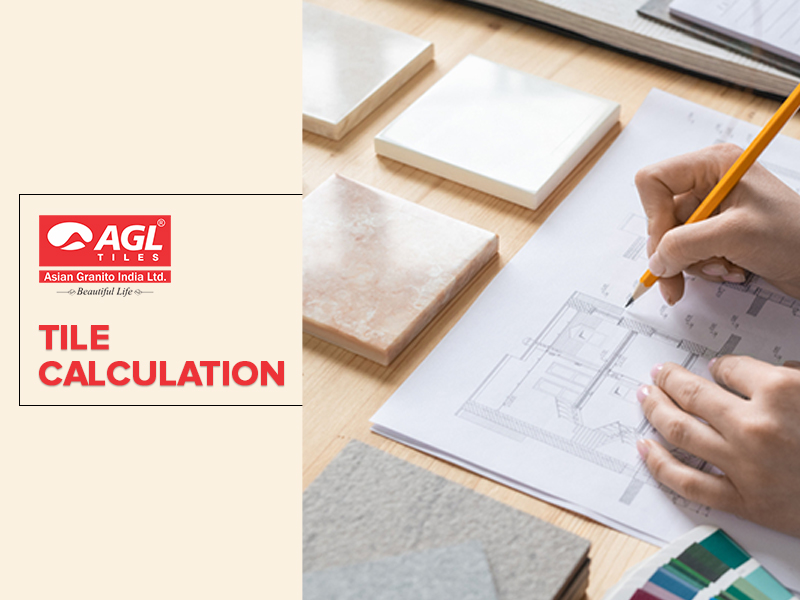Knowing how to calculate square footage is a necessary skill for most home improvement projects. In order to acquire the correct amount of materials for any work, square footage will be an important consideration for flooring. In this post, we'll look at how to use simple calculations to determine how many tiles you'll need for your house remodelling.

Calculating how many tiles you'll need for your project might be difficult. Before beginning any tiling job, whether, on the walls or floors of a large or small space, a sufficient number of tiles must be on hand. The last thing you want to discover midway through your tile job is that you didn't order enough!

Correctly measuring is by far the best way to avoid wasting time and money while tiling a space. It's easy to overthink this process, but it's actually rather simple. With a few calculations and a little help, you can easily obtain the right measurements for the tile you require—don't worry, a math degree isn't required!

In this tiles calculation tutorial, we'll show you how to measure square footage and the tile calculating method for your next project.

So, without further ado, let's get started.

Gather Your Equipments- Whether you're measuring for ceramic floor tiles for your living room or marble kitchen backsplash ideas, your supply list is simple: a measuring tape, a notebook, a pencil, and a calculator.

Taking Measurements: Make sure the space is clear, and nothing is in the way of a straight line with your measuring tape before taking your measurements. Move all furniture to the centre of the room and get rid of any pets that are in your way. Measure the length and breadth of the area, and if it's a circular room, extend your tape measure across the circle's centre and divide it by two.

Calculating Square Feet: Calculate the total size of the space you just measured to determine how many tiles you'll need for a room. That will give you the total square footage in inches for each area where you plan to tile. To get the square feet of the room, multiply the length in feet by the width in feet. L * W = Sq. Ft. Divide the result for a square or rectangle room by 144, and multiply the result by 3.14 for a round room. You will get the square footage of your room.

Calculating the Tile: Aside from the total square footage, you may be interested in the approximate number of tiles needed to cover a given area. Converting the covering area to the number of tiles is a piece of cake! To begin, multiply the length and breadth of one tile in inches to get the area in inches. Finally, divide the space's computed size by the area of one tile. As a consequence, the precise number of tiles necessary for the area is determined.

Including Overage: The 15% overage is also appropriate for tile that will be installed in a space with numerous edges and corners. When more tiles are trimmed to fit tightly around corners, greater waste is to be expected. After determining your ideal overage percentage, multiply the square footage of the space by the overage percentage and add the difference to your total square feet.

If you are looking for wall tiles or floor tiles, may I suggest checking out tiles by AGL? AGL offers a diverse selection of floor and wall tiles in a variety of styles, sizes, and colours for you to choose from.

Original Source:  How to Calculate the Number of Tiles Required?